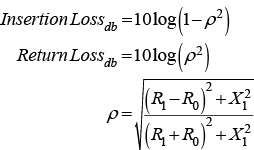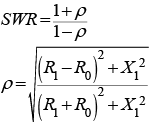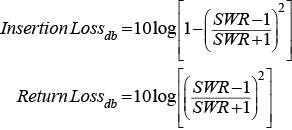## Calculators

 Impedance Mismatch to Power Losses Impedance Mismatch to SWR SWR to Power Losses Z0 + j0 Ω Z0 + j0 Ω SWR to 1 R1 Ω R1 Ω X1 Ω X1 ΩInsertion Loss = dB (S21) SWR = to 1 Insertion Loss = dB (S21) Return Loss = dB (S11) Return Loss = dB (S11)

 NOTE: NOTE: Introduction to scattering parameters (S11 and S21): SWR is always greater than 1 to 1. microwaves101.com/encyclopedias/s-parameters NOTE: This calculator converts the SWR across an impedance mismatch to loss. It does not account for the fact that SWR measured though a lossy (i.e., real) cable will always appear lower than the true SWR.